# Business and Finance Math #1: Future Value of an Annuity Due

Filed under Difficulty: Medium, Finance, HP 12c, TI BA II Plus.

Business and Finance Math is an ongoing series produced by Calcblog to present topics and concepts found in the world of business and finance. Our goal with this series is to provide you with a practical framework for identifying and understanding situations where these business and financial concepts are used. For our first post in this series we present a classic time value of money (TVM) problem involving annuities.

Consider the following situation:

Chuck Moyer, who is currently attending college, has a rich uncle who has decided to put aside some money each year for Chuck so when he graduates he’ll be able to pay off his college loans. Chuck’s uncle places \$5,000 into an investment account earning 6% at the beginning of each year. When Chuck graduates from college in four years, how much money will he receive from his uncle?

Taking the information provided in this question, let’s analyze what type of time value of money (TVM) problem this is. The question describes a series of equal payments of \$5,000 growing at 6% per year for four years. This sounds like a classic annuity problem, but there is one important detail: the \$5,000 payment is invested at the beginning of the year, rather than at the end of the year.

This problem is describing an annuity due, as opposed to an ordinary annuity. The difference between an annuity due and an ordinary annuity is the timing of the cash flows or payments. For an ordinary annuity, the cash flows occur at the end of each year (or time period), while for an annuity due the cash flows are made at the beginning of each time period.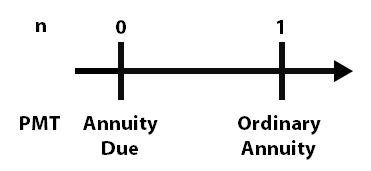Let’s identify the values of the known variables we will be inputting into our calculator to calculate the value of the annuity (move your mouse over the table below to reveal the answers):

N I/Y PV PMT FV
4 6 0 -5000 ?

In this case, we are solving for the amount of money in the investment account after four years (the future value), which is the unknown variable. The number of periods indicated in the question is 4 years, the money in the investment account earns 6% per year, there is no beginning present value so we represent this with a 0, and the amount of money deposited at the beginning of each year is \$5,000. The negative sign in front of the \$5,000 for the payment is a convention used by financial calculators. Think of it this way: Chuck’s uncle is depositing \$5,000 a year (a cash outflow) to receive it back in the future (the future value, which is positive). The timeline for the cash flows is as follows: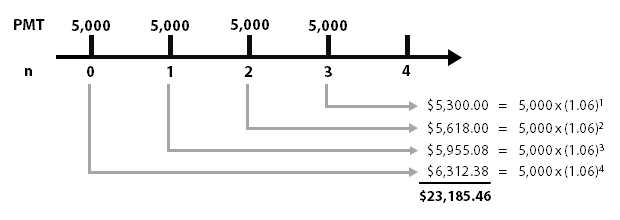Let’s prepare our calculator to solve this problem.

### TI BA II Plus Version

The first step is to clear your calculator’s memory to ensure there is no information from a previous TVM problem. Press the 2nd key and then the button. This selects the “CLR TVM” command and clears out any data you entered using the calculator’s TVM keys.

The second step is to temporarily adjust your calculator’s annuity mode. By default your TI BA II Plus should be set to “end” mode, which means any annuity cash flows occur at the end of each period. Since we are solving an annuity due, we need to change the timing of the cash flows. Press 2nd . If you see the word “END”, press 2nd to change the mode to “BGN.” Otherwise, just exit this screen.

Now you’re ready to solve the problem. Enter the following on your calculator:

• 4
• 6
• 0
• 5000

You should receive a future value of 23,185.46. When Chuck graduates in four years, he will receive \$23,185.46 from his wealthy uncle.

The last step is to prepare your calculator for next time. Clear your TVM again, then press 2nd and then 2nd to change the calculator’s annuity mode back to “END.”

### HP 12C Version

Clear your calculator’s memory by pressing f and then key located left of the key.

Change the calculator’s annuity mode from END to BEG so cash flows are measured at the beginning of each period rather than at the end. To do this press g . Note if you see the word “BEGIN” on your calculator screen you can skip this step.

Now you’re ready to solve the problem. Enter the following on your calculator:

• 4
• 6
• 0
• 5000

Your calculator should display 23,185.46. When Chuck graduates college, his uncle will be able to give him \$23,185.46 to pay off his debts.

Clear your TVM memory by repeating the first step and change your mode back to END by pressing g .

### Annuity Due Calculations

The formula to calculate an ordinary annuity is as follows: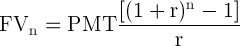Only one slight adjustment needs to be made to this formula to change this formula to calculate an annuity due. An annuity due can be computed by first calculating an ordinary annuity, then multiplying it by 1 plus the interest rate (1 +r):

Annuity Due = Ordinary Annuity Value x (1 + r)

Our modified annuity due formula is: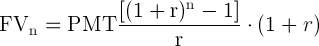Taking the variables from the question above, you could also solve for the solution using our modified annuity due formula: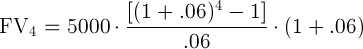Reviewing the calculator steps, we can eliminate the initial step of switching from End to Begin mode. Simply solve for an ordinary annuity using the same variables, then multiple your answer by 1.06 (1 plus the interest rate of 6%).

Annuity Due = 21,873.08 x (1.00 + 0.06)

Annuity Due = \$23,185.46

Why does this work? In an ordinary annuity, the cash flows occur at the end of each period. As we already discussed, payments occur at the beginning of each period for annuities due. If we solve for an ordinary annuity when trying to calculate an annuity due, the payments must be compounded for an extra period. This is because the ordinary annuity does not account for that first cash flow/payment that occurs immediately at the beginning in an annuity due. By multiplying the ordinary annuity formula by (1 + r) we compensate for that additional compounding to calculate the annuity value.

### 3 Responses to Business and Finance Math #1: Future Value of an Annuity Due

1.Marcos Guimaraes

Do you have the same explanation for the effect of annuity due and ordinary annuity in NPV calculation of given cash flow?
I checked in my HP12 C using begin and end however I am getting the same value regarding the annuity mode of payment.

Regards, Marcos Guimaraes.